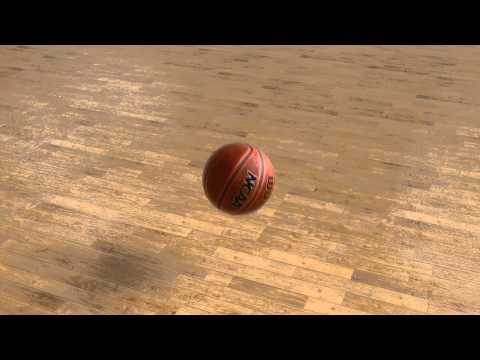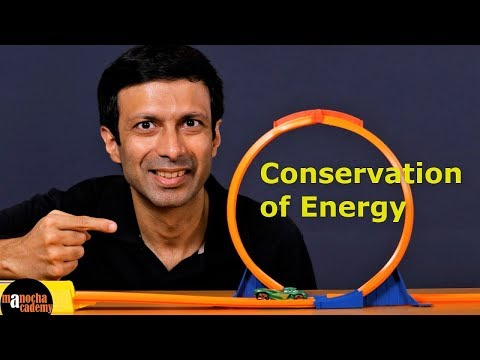Join game?
Enter PIN:

# Roller coaster Physics

2.7
10 questions
English
A set of rules that run the roller-coaster
1## What kind of energy is in bouncing ball?

Mechanical Energy

Elastic energy

Kinetic energy Only

Potential Energy only

###13% got this right

The ball is in motion so it's have mechanical energy (both K.E and P.E).
2## What form of energy is stored in the rubber band?

Elastic Potential energy

Rotational kinetic energy

Gravitational Energy

Kinetic Energy

### 64% got this right

The stretch rubber band has stored Potential Energy in it.
3## What form of energy of the car at start?

Kinetic Energy

Fuel Energy

Potential Energy

Stored Energy

###29% got this right

The Car has Kinetic energy. The energy of motion.
4## The moving car goes into loop, what form of energy does it have?

Kinetic Energy and Potential Energy

K.E and P.E cancelled each other

Kinetic energy Only

Potential Energy only

### 63% got this right

The car has both Kinetic and Potential energies.
5## What happened when car reaches the top of the loop, what form energy does it has?

Both Kinetic and Potential Energy

Gravitational Potential Energy only

Maximum Potential Energy

Minimum Kinetic energy

###20% got this right

The car has both Kinetic and Potential energies. Since the speed of the car is not zero.
6## When car exits the loop what kind of energy does it have?

Maximum Kinetic Energy

Maximum Potential Energy

Minimum Kinetic energy

Both Kinetic & Potential Energy

### 39% got this right

The car only has Kinetic energy due to its motion.
7## When car hits the block and come to stop what form of energy is not involved?

Potential Energy

Heat Energy

Kinetic Energy

Sound Energy

###28% got this right

Heat and sound energy will produce in this collision and some kinetic energy is transferred to block.
8## In this whole experiment what physics law is conserved?

Law of Conservation of energy

Law of conservation of mass

Law of conservation of momentum

Law of conservation of speed

###32% got this right

Law of conservation of energy: the amount of energy is conserved but energy changes from one form to another form.
9## What force is needed for circular motion?

Centripetal Force

Centrifugal Force

Normal force

Weight of the object

###29% got this right

A centripetal force is a force that makes a body follow a curved path and directed inward toward the center of rotation
10## What kind of motion the car is in when it goes around the loop?

Circular motion

Periodic motion

Rotatory Motion

Curvilinear motion

### 49% got this right

Circular motion is a special case of curvilinear motion in which the radius of rotation remains constant

## Similar quizzes3.63.53.6